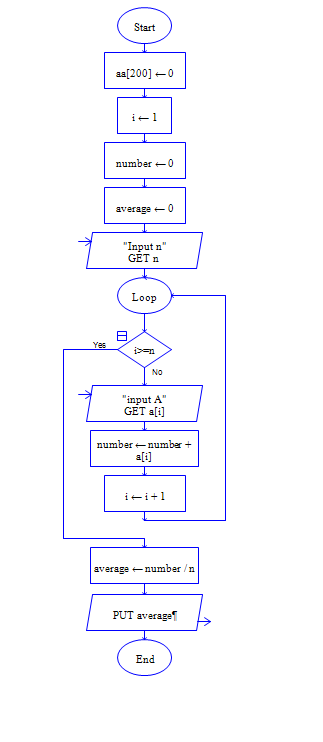# C Program to find the sum and average of array Elements

Get array size n and n elements of array, then compute sum and average of the elements.

Sample Input 1:

5 5 7 9 3 1

Sample Output 1:

25 5.0

#### Flow Chart DesignStrongly recommended to Solve it on your own, Don't directly go to the solution given below.

#include<stdio.h> int main() { //write your code here }

#### Program or Solution

``` #include <stdio.h> #include <stdlib.h> int main(void) { int *a,n,i,sum=0,avg; printf("Enter size of array:"); scanf("%d",&n); a=malloc(sizeof(int)*n); printf("Enter %d Elements:",n); for(i=0;i<n;i++) { scanf("%d",&a[i]); } for(i=0;i<n;i++) { sum=sum+a[i]; } avg=sum/n; printf("\nSum of array:%d \nAverage of Array:%d",sum,avg); return 0; } ```

#### Program Explanation

i is initialized to 0 and incremented by 1 at each iteration of both the for loops.

First for loop reads n input numbers from user and stores them in array a[] from location 0 to n-1

Second for loop adds all the n elements of array located from 0 to n-1

Finally compute average using avg=sum/n statement and prints average avg using printf statement.